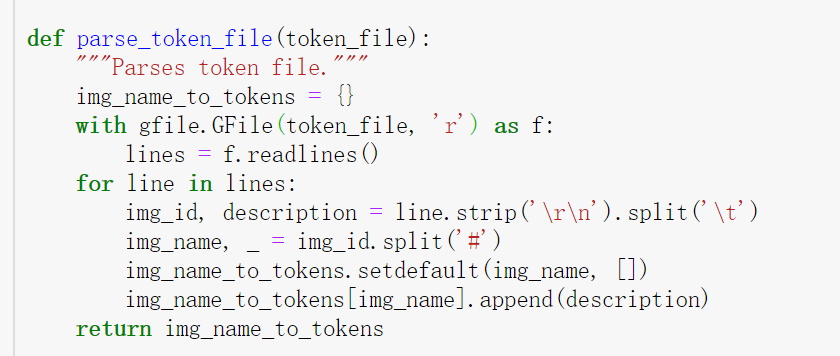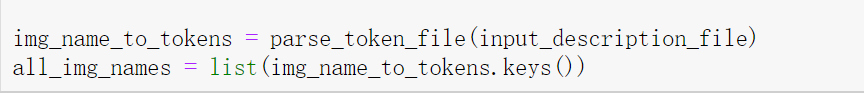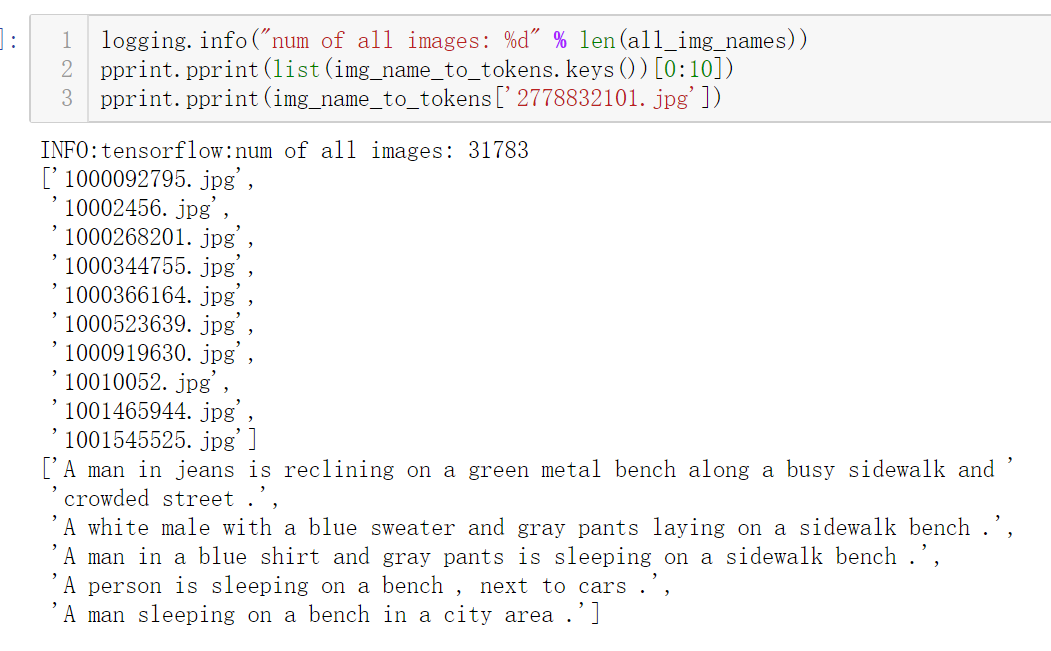2018-09-06 17:26:18 sy20173081277 阅读数 4657
• ###### MATLAB图像处理

全面系统的学习MATLAB在图像处理中的应用

19880 人正在学习 去看看 魏伟

首先，基于图像的识别，分类等操作，具有无接触、高精度的特点，尤其在活体检测、识别、分类等过程中这种无接触的方式适用性极强。

然而到目前为止，在图像识别领域的每一个重大的突破无一不都是用到了卷积神经网络。卷积神经网络CNN以及众多以此衍生出来的网络模型，可以直接将图像数据作为输入，不仅无需人工对图像进行预处理和额外的特征抽取等复杂操作，而且以其特有的细粒度特征提取方式，使得对图像的处理达到了几近人力的水平。这可能也是CNN为何这么火的原因吧。

无法预知以后会不会有新的网络模型可以取而代之。

2019-09-25 15:33:49 weixin_43991178 阅读数 83
• ###### MATLAB图像处理

全面系统的学习MATLAB在图像处理中的应用

19880 人正在学习 去看看 魏伟

# 针孔相机

SLAM中采用的相机模型大多基于针孔相机模型，在说明针孔相机模型的投影关系之前，先明确需要用到的几个坐标系下用一个空间点的不同坐标表示：

-世界坐标系$w$：空间点在世界坐标系下的坐标表示为$\bm{P}^w=\left[\begin{array}{ccc}X & Y & Z \end{array}\right]^T$

• 相机坐标系$c$：空间点在相机坐标系下的坐标表示为$\bm{p}^c=\left[\begin{array}{ccc}x & y & z \end{array}\right]^T$
• 像素坐标系$p$：空间点在像素坐标系下的坐标表示为$\bm{u}^p=\left[\begin{array}{cc}u & v \end{array}\right]^T$

$\bm{p}=\bm{T}\bm{P}$

\left\{\begin{aligned} x'&=\frac{f}{z}x\\ y'&=\frac{f}{z}y \end{aligned} \right.

\left\{\begin{aligned} u&=\alpha\frac{f}{z}x+c_x\\ v&=\beta\frac{f}{z}y+c_y \end{aligned} \right.
$\alpha f=f_x$$\beta f=f_y$，同时将上式写成齐次矩阵形式为：
$\frac{1}{z}\left[\begin{array}{c}u\\v\\1\end{array}\right]=\underbrace{\left[\begin{matrix} f_x & 0 & c_x\\ 0 & f_y & c_y\\ 0 & 0 & 1 \end{matrix}\right]}_{\bm{K}}\left[\begin{array}{c}x\\y\\z\end{array}\right]$

## 畸变模型

### 径向畸变模型

\left\{\begin{aligned} x_{distorted}&=x\left(1+k_1r^2+k_2r^4\right)\\ y_{distorted}&=y\left(1+k_1r^2+k_2r^4\right)\\ r^2&=x^2+y^2 \end{aligned} \right.

### 切向畸变模型

\left\{\begin{aligned} x_{distorted}&=x+\left[2p_1xy+p_2\left(r^2+2x^2\right)\right]\\ y_{distorted}&=y+\left[p_1\left(r^2+2y^2\right)+2p_2xy\right]\\ r^2&=x^2+y^2 \end{aligned} \right.

### 联合畸变模型

\left\{\begin{aligned} x_{distorted}&=x\left(1+k_1r^2+k_2r^4\right)+\left[2p_1xy+p_2\left(r^2+2x^2\right)\right]\\ y_{distorted}&=y\left(1+k_1r^2+k_2r^4\right)+\left[p_1\left(r^2+2y^2\right)+2p_2xy\right]\\ r^2&=x^2+y^2 \end{aligned} \right.

## 畸变矫正方法

\begin{aligned} &\left\{\begin{aligned} &x\left(1+k_1r^2+k_2r^4\right)=x_{distorted}-\left[2p_1xy+p_2\left(r^2+2x^2\right)\right]\\ &y\left(1+k_1r^2+k_2r^4\right)=y_{distorted}-\left[p_1\left(r^2+2y^2\right)+2p_2xy\right]\\ \end{aligned}\right.\\ &\Rightarrow\left\{\begin{aligned} x&=\frac{x_{distorted}-\left[2p_1xy+p_2\left(r^2+2x^2\right)\right]}{\left(1+k_1r^2+k_2r^4\right)}\\ y&=\frac{y_{distorted}-\left[p_1\left(r^2+2y^2\right)+2p_2xy\right]}{\left(1+k_1r^2+k_2r^4\right)}\\ \end{aligned}\right.\\ \end{aligned}

/*
* @Description:
* @Author: Yuntian Li
* @Github: https://github.com/yuntinali91
* @Date: 2019-09-24 14:28:08
* @LastEditors: Yuntian Li
* @LastEditTime: 2019-09-25 15:13:20
*/
#include <iostream>
#include <opencv2/opencv.hpp>
#include <Eigen/Dense>

using namespace std;

void distortion(const Eigen::Vector2d& u_u, Eigen::Vector2d& u_d,
const Eigen::Matrix3d& K, const Eigen::Vector4d& D);
void undistortion(const Eigen::Vector2d& u_u, Eigen::Vector2d& u_d,
const Eigen::Matrix3d& K, const Eigen::Vector4d& D, const double tolerance);

int main(int argc, char** argv){
// distortion coefficients
Eigen::Vector4d D{-2.917e-1, 8.228e-2, 5.333e-5, -1.578e-4};
// intrinsic matrix
Eigen::Matrix3d K;
K << 4.616e2, 0, 3.63e2,
0, 4.603e2, 2.481e2,
0, 0, 1;

Eigen::Vector2d u{40., 50.};
Eigen::Vector2d u_u, u_d;

distortion(u, u_d, K, D);
cout << "Distorted point coordinates are:" << endl << u_d << endl;

double tolerance(1e-4);
undistortion(u_d, u_u, K, D, tolerance);
cout << "Undistorted point coordinates are: " << endl << u_u << endl;

return 0;
}

void distortion(const Eigen::Vector2d& u_u, Eigen::Vector2d& u_d,
const Eigen::Matrix3d& K, const Eigen::Vector4d& D){
// lift from pixel plane to normalized plane
Eigen::Vector3d p_u;
Eigen::Vector3d u_u_homo{0., 0., 1.};

p_u = K.inverse() * u_u_homo;
// apply distortion
double x_u, y_u;
x_u = p_u; y_u = p_u;

double k1, k2; // radial distortion coefficients
double p1, p2; // tangent distortion coefficients
k1 = D; k2 = D;
p1 = D; p2 = D;

double x_u2, y_u2, xy_u, r2;
x_u2 = x_u * x_u;
y_u2 = y_u * y_u;
xy_u = x_u * y_u;
r2 = x_u2 + y_u2;

Eigen::Vector3d p_d;
p_d = x_u * (1 + k1 * r2 + k2 * r2 * r2) + (2 * p1 * xy_u + p2 * (r2 + 2 * x_u2));
p_d = y_u * (1 + k1 * r2 + k2 * r2 * r2) + (2 * p2 * xy_u + p1 * (r2 + 2 * y_u2));
p_d = 1.;
// project from normalized plane to normalized plane
}

void undistortion(const Eigen::Vector2d& u_d, Eigen::Vector2d& u_u,
const Eigen::Matrix3d& K, const Eigen::Vector4d& D, const double tolerance){
// lift from pixel plane to normalized plane
Eigen::Vector3d p_d;
Eigen::Vector3d u_d_homo{0., 0., 1.};

p_d = K.inverse() * u_d_homo;

// recursive undistortion
double k1, k2; // radial distortion coefficients
double p1, p2; // tangent distortion coefficients
k1 = D; k2 = D;
p1 = D; p2 = D;

double x_d, y_d;
x_d = p_d; y_d = p_d;

Eigen::Vector3d p_u;
p_u = 1.0;

int count(0), conv_count(0);

while (1){

count++;
double x_d2, y_d2, xy_d, r2;
x_d2 = x_d * x_d;
y_d2 = y_d * y_d;
xy_d = x_d * y_d;
r2 = x_d2 + y_d2;

p_u = (p_d - (2 * p1 * xy_d + p2 * (r2 + 2 * x_d2))) / (1 + k1 * r2 + k2 * r2 * r2);
p_u = (p_d - (2 * p2 * xy_d + p2 * (r2 + 2 * y_d2))) / (1 + k1 * r2 + k2 * r2 * r2);

// project from normalized plane to pixel plane
cout << "Iterative times: " << count << "  Estimation: " << u_u.transpose() << endl;

// termination criteria
double u_err, v_err;
u_err = abs(K(0, 0) * (p_u - x_d));
v_err = abs(K(1, 1) * (p_u - y_d));
if ( u_err < tolerance && v_err < tolerance) conv_count++;

if (conv_count > 3){
cout << "Total Iterative times: " << count << endl
<< "Final error: " << u_err << " pixels in x and " << v_err << " pixels in y." << endl
<< "Final estimation: " << u_u.transpose() << endl;
break;
}

x_d = p_u; y_d = p_u;

}
}


# 鱼眼相机模型

## Kannala畸变模型\begin{aligned} X_n^c &= X^c / Z^c\\ Y_n^c &= Y^c / Z^c \end{aligned} \tag{1}

\begin{aligned} r^c& = \sqrt{{X_n^c}^2+{X_n^c}^2}\\ \theta&=atan(r^c/1)=atan(r^c) \end{aligned} \tag{2}

\left\{ \begin{aligned} \frac{X_n^c}{r^c}&=\frac{x}{r^i}\\ \frac{Y_n^c}{r^c}&=\frac{y}{r^i} \end{aligned} \right. \Rightarrow \left\{ \begin{aligned} x &= \frac{f\theta}{r^c}X_n^c\\ y &= \frac{f\theta}{r^c}Y_n^c \end{aligned} \right. \Rightarrow \left\{ \begin{aligned} x &= \frac{\theta}{r^c}X_n^c\\ y &= \frac{\theta}{r^c}Y_n^c \end{aligned} \right. \tag{3}

$\theta_d=\theta+k_1\theta^3+k_2\theta^5+k_3\theta^7+k_4\theta^9 \tag{4}$

## 鱼眼相机畸变校正

\begin{aligned} X_1^c&=R\sin(\theta)\cos(\varphi)\\ Y_1^c&=R\sin(\theta)\sin(\varphi)\\ Z_1^c&=R\cos(\theta) \end{aligned}

• $\varphi$求解
成像面上夹角$\varphi_1$可以用像点坐标表示为：$\tan(\varphi_1)=y/x$。由于入射光线和出射光线必定在同一个入射面内，有$\varphi=\varphi_1$，因此未畸变的极坐标参数$\varphi$为：
$\varphi=\tan(y/x)$
• $\theta$求解
畸变后的入射角$\theta_d$可以用像点坐标表示为$\theta=\sqrt{(x^2+y^2)}$$\theta$角可通过牛顿迭代求解式（4）所表示的多项式方程获得，令：
$f(\theta)=\theta+k_1\theta^3+k_2\theta^5+k_3\theta^7+k_4\theta^9 - \theta_d$
则根据牛顿迭代有：
$\theta_{k+1}=\theta_k-\frac{f(\theta)}{f'(\theta)}$

2019-04-29 15:14:42 weixin_43002202 阅读数 476
• ###### MATLAB图像处理

全面系统的学习MATLAB在图像处理中的应用

19880 人正在学习 去看看 魏伟

1、图像编解码处理

• JPEG: 应用最广泛的图片格式之一，它采用一种特殊的有损压缩算法，将不易被人眼察觉的图像颜色删除，从而达到较大的压缩比（可达到2:1甚至40:1），所以“身材娇小，容貌姣好”，特别受网络青睐 ,JPEG的文件格式一般有两种文件扩展名：.jpg和.jpeg，这两种扩展名的实质是相同的，我们可以把.jpg的文件改名为.jpeg，而对文件本身不会有任何影响。
• GIF：分为静态GIF和动画GIF两种，支持透明背景图像，适用于多种操作系统，“体型”很小，网上很多小动画都是GIF格式。其实GIF是将多幅图像保存为一个图像文件，从而形成动画,所以归根到底GIF仍然是图片文件格式。
• PNG: 与JPG格式类似,网页中有很多图片都是这种格式，压缩比高于GIF，支持图像透明，可以利用Alpha通道调节图像的透明度,因此.png图像是一个四通道的图像。

API1：tf.gfile.FastGFile(path,decodestyle) 图片读取

1、函数功能：实现从存储的文件当中对图片的读取。

2、函数参数：

• path：图片所在路径
• decodestyle:图片的解码方式。(‘r’:UTF-8编码; ‘rb’:非UTF-8编码)

UTF 是 Unicode Transformation Format 的缩写，意思是“Unicode转换格式”，后面的数字表明至少使用多少个比特位（Bit）来存储字符。是一种编码方式，UFT-8是Unicode编码方式的一种。

• API2：tf.image.decode_png(contents, channels=None, name=None) .png图片的解码函数

1、函数功能：TensorFlow提供了decode_png()函数将.png格式的图像解码从而得到图像对应的三维矩阵,数据类型为uint8。

2、函数参数：

• contents: string”类型的张量,使用png编码格式的图片
• channels: 默认是None,表示解码图像的颜色通道数。
• 3、其他解码函数

• .jpg.jpeg: tf.image.decode_jpeg()
• .gif: tf.image.decode_gif()
•
• name: A name for the operation (optional).

API3：tf.image.encode_png(image)

1、函数功能：表示一张图片的三维矩阵重新按照png格式编码并存入文件中

2、函数参数：

• image: sshape为3的Tensor

3、其他编码函数

• .jpg.jpeg: tf.image.encode_jpeg()

import matplotlib.pyplot as plt
import tensorflow as tf

# 读取原始的图像,实现对图片的读取，读取到内存当中

with tf.Session() as sess:

img_after_decode = tf.image.decode_png(image)

# 输出解码之后的三维矩阵，并调用pyplot工具可视化得到的图像

print(img_after_decode.eval())

plt.imshow(img_after_decode.eval())#负责处理
plt.show()#负责执行

# 这一句是为了方便后续的样例程序对图像进行处理
# img_after_decode = tf.image.convert_image_dtype(img_after_decode,dtype = tf.float32)

# TensorFlow提供了encode_png()函数将解码后的图像进行再编码
# 表示一张图片的三维矩阵重新按照png格式编码并存入文件中。在资源管理器中打开这张图像，可以得到和原始图像一样的图像
encode_image = tf.image.encode_png(img_after_decode)
with tf.gfile.GFile("cat.png", "wb") as f:
f.write(encode_image.eval())

2、翻转图像

API4 ： tf.image.random_flip_left_right( image,seed=None) 随机左右翻转函数

1、函数功能：按水平 (从左向右)方向 随机翻转图像，将图像左右翻转的概率为0.5，即输出的图像有50％的可能性是上左右翻转的，否则就输出原图．

2、函数参数：

• image：形状为[height, width, channels]的三维张量.
• seed：一个Python整数,用于创建一个随机种子.

图像固定左右翻转: lipped2 = tf.image.flip_left_right(image)

import matplotlib.pyplot as plt
import tensorflow as tf

with tf.Session() as sess:
#decode
img_after_decode = tf.image.decode_png(image)
# 以一定概率左右翻转图片。
# 函数原型为random_flip_left_right(image,seed)
flipped = tf.image.random_flip_left_right(img_after_decode)

# 用pyplot工具显示
plt.imshow(flipped.eval())
plt.show()

3、图像色彩调整

* 亮度调整

API 5：tf.image.random_brightness(image, max_delta,seed=None) 随机调整亮度函数

1、函数功能：在某范围[-max_delta, max_delta)中随机选取的delta，来随机调整图片亮度,公式为：????????=????????+?????newimage=oldimage+delta

2、函数参数：

• image： 一个图像.
• max_delta：float型数据,必须是非负的

tf.image.adjust_brightness(image,delta)

#随机调整亮度 tf.image.random_brightness

import matplotlib.pyplot as plt
import tensorflow as tf

with tf.Session() as sess:
img_after_decode = tf.image.decode_png(image)

#函数原型random_brightness(image,max_delta,seed)
#max_delta的值不能为负，函数会在[-max_delta，max_delta]值之间随机调整图像的亮度

# 用pyplot工具显示
plt.show()


* 对比度调整

API 6：tf.image.random_contrast(image,lower,upper,seed=None) 随机对比度调整函数

1、函数功能：通过在某范围[lower,upper)中随机选取调整对比度因子contrast_factor，以随机调整图像的对比度. 改变原图片的对比度，公式为(?−????)∗??????????????+????(?−????)∗??????????????+????(x−mean)∗contrastfactor+mean(x−mean)∗contrastfactor+mean, 其中的x为原输入，mean为每个通道的均值。

2、函数参数：

• image：具有3个或更多维度的图像张量
• lower：浮点型,随机对比因子的下限.
• upper：浮点型,随机对比因子的上限.

tf.image.adjust_contrast(images,contrast_factor)

#随机调整对比度tf.image.random_contrast
import matplotlib.pyplot as plt
import tensorflow as tf

with tf.Session() as sess:
img_after_decode = tf.image.decode_png(image)

#函数原型random_contrast(image,lower,upper,seed)
#函数会在[lower upper]之间随机调整图像的对比度
#但要注意参数lower和upper都不能为负

plt.show()


* 色相调整

1、函数功能：改变原图像的色彩(hue)，本函数将原RGB图像转换到HSV空间后，在hue通道上加上delta后，再转换回RGB空间。

HSV(Hue, Saturation, Value)是根据颜色的直观特性由A. R. Smith在1978年创建的一种颜色空间,这个模型中颜色的参数分别是：色调（H），饱和度（S），明度（V）

RGB和HSV转换

2、函数参数：

• image：RGB图像或图像
• delta：浮点型,要添加多少色相通道.

3、注意：

tf.image.random_hue(image, max_delta)

#固定调整色相adjusted_hue

import matplotlib.pyplot as plt
import tensorflow as tf

with tf.Session() as sess:
img_after_decode = tf.image.decode_jpeg(image)

plt.show()


* 饱和度调整

1、函数功能：将RGB图像转换为浮点表示形式,将其转换为HSV,向饱和通道添加偏移量,再转换回RGB

2、函数参数：

• image：RGB图像或图像,最后尺寸的大小必须为3
• saturation_factor：浮点型,因子乘以饱和度

tf.image.random_saturation(image, lower, upper,seed)在[lower, upper]的范围随机调整图的饱和度。

#固定调整饱和度tf.image.adjust_saturation
import matplotlib.pyplot as plt
import tensorflow as tf

with tf.Session() as sess:
img_after_decode = tf.image.decode_jpeg(image)

#将图片的饱和度调整-6。
#将图片的饱和度调整+6。

plt.show()

5、调整图像大小

*保留全部图像信息的缩放

API 9：tf.image.resize_images(image，size， method)

1、函数功能：使用指定的调整方法method调整images尺寸为为size的大小.

2、函数参数：

• image：需要改变尺寸的图像
• size： 改变之后图像的大小
• method： 用于表示改变图像过程用的差值方法。有四种取值分别对应不同的调整方法
  0：双线性插值法。
1：最近邻居法。
2：双三次插值法。
3：面积插值法。

四种方法有细微的差别，但是如果没有特别的要求，可以认为结果是相同的,一般采用双线插值法。

import matplotlib.pyplot as plt
import tensorflow as tf
import numpy as np

with tf.Session() as sess:
img_after_decode = tf.image.decode_png(image)

#函数原型resize_images(images,size,method,align_corners)，原图大小895x560

resized0 = tf.image.resize_images(img_after_decode, [300, 300], method=0)
resized1 = tf.image.resize_images(img_after_decode, [300, 300], method=1)
resized2 = tf.image.resize_images(img_after_decode, [300, 300], method=2)
resized3 = tf.image.resize_images(img_after_decode, [300, 300], method=3)

print(resized.dtype)
#打印的信息<dtype: 'uint8'>

# 从print的结果看出经由resize_images()函数处理图片后返回的数据是float32格式的，
# 所以需要转换成uint8才能正确打印图片，这里使用np.asarray()存储了转换的结果
resized0 = np.asarray(resized0.eval(), dtype="uint8")
resized1 = np.asarray(resized1.eval(), dtype="uint8")
resized2 = np.asarray(resized2.eval(), dtype="uint8")
resized3 = np.asarray(resized3.eval(), dtype="uint8")

plt.imshow(resized0)
plt.show()
plt.imshow(resized1)
plt.show()
plt.imshow(resized2)
plt.show()
plt.imshow(resized3)
plt.show()

*图像裁剪或填充（中心位置）

1、函数功能：实现了裁剪或者填充的功能，是第四节中用到的裁剪函数，在使用时会会传入的图像数据制定一个目标大小

如果原始图像的大小小于目标大小，则函数会在原始图像的四周进行全0填充，以达到目标大小；

2、函数参数：

• image：需要改变尺寸的图像
• target_height：目标高度.
• target_width：目标宽度.

#随机裁剪填充函数tf.image.resize_image_with_crop_or_pad()

import matplotlib.pyplot as plt
import tensorflow as tf

with tf.Session() as sess:
img_after_decode = tf.image.decode_png(image)

#裁剪图像
#填充图像

#用pyplot显示结果
plt.imshow(croped.eval())
plt.show()
plt.show()

*按比例裁剪图像

API 11：tf.image.central_crop(image,central_fraction)

1、函数功能：以原始图像的中心为中心，按照传递进来的比例参数将图像进行裁剪，保留中心区域。

2、函数参数：

• image：需要改变尺寸的图像
• central_fraction：float类型，取值在(0,1]内,要裁剪的大小部分的比率

#按比例调整图像大小函数 tf.image.central_crop()
import matplotlib.pyplot as plt
import tensorflow as tf

with tf.Session() as sess:
img_after_decode = tf.image.decode_png(image)

# 函数原型central_crop(image,central_fraction)
#参数central_fraction是裁剪比例

central_cropped = tf.image.central_crop(img_after_decode, 0.4)

plt.imshow(central_cropped.eval())
plt.show()

*指定位置裁剪、填充图像

API 12.1：tf.image.crop_to_bounding_box(image,offset_height,offset_width,target_height,target_width)

tf.image.crop_to_bounding_box()

• offset_height：表示目标图像的左上角距离原始顶部边的行数

• offset_width：表示目标图像的左上角距离原始图像左侧边的列数

• target_height,target_width：表示裁剪后的目标图像大小

tf.image.pad_to_bounding_box()

• offset_height：表示在图像的顶部添加全0填充的行数

• offset_width：表示在图像的左侧添加全0填充的列数

• target_height,target_width：表示填充后的目标图像大小

import matplotlib.pyplot as plt
import tensorflow as tf

with tf.Session() as sess:
img_after_decode = tf.image.decode_png(image)

# 函数原型
# crop_to_bounding_box(image,offset_height,offset_width,target_height,target_width)
croped = tf.image.crop_to_bounding_box(img_after_decode, 100, 100, 300, 300)

plt.imshow(croped.eval())
plt.show()
plt.show()


6、图像的标注框

*矩形标注框

API 13：tf.image.draw_bounding_boxes(images,boxes,name=None)

1、函数功能：在一批图像上绘制边界框。

在图像像素之上绘制由位置指定的边界框boxes，可以指定一个或多个boxes。

2、函数参数：

• image：四维的 Tensor，形状[batch, height, width, depth]，一批图片。
• boxes：类型为float32，shape为三维的Tensor。

API 14：tf.expand_dims(input, dim, name=None)

1、函数功能：实现给定一个input，给input增加一个维度。
tf.image.draw_bounding_boxes()函数要求输入的图像数据是是实数型，并且输入是一个batch的数据，因此是一个四维矩阵，所以要增加一个维度

2、函数参数：

• input：输入的Tensor
• dim：增加的维度，从0开始插入

import matplotlib.pyplot as plt
import tensorflow as tf

with tf.Session() as sess:
img_after_decode = tf.image.decode_png(image)

# tf.expand_dims()将矩阵转化为为四维矩阵，函数原型expand_dims(input,axis,name,dim)
# tf.image.convert_image_dtype()将数据类型转化为实数型
batched = tf.expand_dims(tf.image.convert_image_dtype(img_after_decode, tf.float32), 0)

# 定义边框的坐标系数
boxes = tf.constant([[[0.05, 0.05, 0.9, 0.7], [0.20, 0.3, 0.5, 0.5]]])

# 绘制边框，函数原型draw_bounding_boxes(images,boxes,name)
image_boxed = tf.image.draw_bounding_boxes(batched, boxes)

# draw_bounding_boxes()函数处理的是一个batch的图片，如果此处给imshow()函数
# 传入image_boxed参数会造成报错(Invalid dimensions for image data)
plt.imshow(image_boxed.eval())
plt.show()


*随机变形的边界框

API 15：tf.image.sample_distorted_bounding_box(

image_size,
bounding_boxes,
seed=None,
seed2=None,
min_object_covered=None,
aspect_ratio_range=None,
area_range=None,
max_attempts=None,
use_image_if_no_bounding_boxes=None,
name=None

)

1、函数功能：此函数为图像生成单个随机变形的边界框。函数输出的是可用于配合tf.slice()函数裁剪原始图像；还可以配合tf.image.draw_bounding_boxes()产生随机标注框

2、函数参数：

• image_size：一个Tensor,必须是下列类型之一：uint8,int8,int16,int32,int64,是1维的,并且包含[height, width, channels]

• bounding_boxes：一个float32类型的三维Tensor,形状为[batch, N, 4],描述与图像相关的Ñ个边界框。

例如bounding_boxes = tf.constant([[[0.05, 0.05, 0.9, 0.7], [0.20, 0.3, 0.5, 0.5]]])，它的形状就是[1,2,4]

3、返回值：

• begin，size： 用于 tf.slice() 剪裁图像，作为输入的参数，随机裁剪

• bboxes： 可以用于 tf.image.draw_bounding_boxes 函数来画出边界框,bboxes形状是固定的[1,1,4]，因此最终只有一个标注边框的输出

tf.image.sample_distorted_bounding_box()函数的返回值具有随机性，因此标注的框和裁剪出来的图片也都是随机的，所以每次得到的结果也不相同。

import matplotlib.pyplot as plt
import tensorflow as tf

with tf.Session() as sess:
img_after_decode = tf.image.decode_png(image)
boxes = tf.constant([[[0.05, 0.05, 0.9, 0.7], [0.20, 0.3, 0.5, 0.5]]])

#函数原型
#sample_distorted_bounding_box(image_size,bounding_boxes,seed,seed2,min_object_covered,
# aspect_ratio_range,area_range,max_attempts,use_image_if_no_bounding_boxes,name)

begin, size, bounding_box = tf.image.sample_distorted_bounding_box(tf.shape(img_after_decode), bounding_boxes=boxes)

batched = tf.expand_dims(tf.image.convert_image_dtype(img_after_decode, tf.float32), 0)
image_boxed = tf.image.draw_bounding_boxes(batched, bounding_box)

#slice()函数原型slice(input_,begin,size,name)
sliced_image = tf.slice(img_after_decode,begin,size)

plt.imshow(image_boxed.eval())
plt.show()
plt.imshow(sliced_image.eval())
plt.show()


2018-12-01 11:38:50 qq_38662930 阅读数 80
• ###### MATLAB图像处理

全面系统的学习MATLAB在图像处理中的应用

19880 人正在学习 去看看 魏伟

# keras常用语法

Sequential模型
Keras一般用Sequential模型做为搭建神经网络的开始，本节简要论述Sequential模型接口的主要使用方法。

pop(self)

compile(self,optimizer,loss,metrics=None,sample_weight_mode=None)

• optimizer：字符串（预定义优化器名）或优化器对象，参考优化器。
• loss：字符串（预定义损失函数名）或目标函数，参考损失函数。
• metrics：列表，包含评估模型在训练和测试时的网络性能的指标，典型用法是 metrics=[‘accuracy’]。
• sample_weight_mode：如果你需要按时间步为样本赋权（2D权矩阵），将该值设
为“temporal”。默认为“None”，代表按样本赋权（1D权）。
• kwargs：使用TensorFlow作为后端请忽略该参数，若使用Theano作为后端，kwargs的 值将会传递给K.function。

model=Sequential()
model.compile(optimizer='rmsprop',
loss='categorical_crossentropy',metrics=['accuracy'])



categorical_crossentroy，各种损失函数的含义在后面章节中会提高。

fit(self,x,y,batch_size=32,epochs=10,verbose=1,callbacks=None,validation_split=0.0,val）
.

• x：输入数据。如果模型只有一个输入，那么x的类型是numpyarray，如果模型有多
个输入，那么x的类型应当为list，list的元素是对应于各个输入的numpyarray。
• y：标签向量，numpyarray类型。
• batch_size：整数，指定进行梯度下降时每个batch包含的样本数。训练时一个batch的
样本会被计算一次梯度下降，使目标函数优化一步。
• epochs：训练的轮数，每个epoch会把训练集轮一遍。
• verbose：日志显示，0为不在标准输出流输出日志信息，1为输出进度条记录，2为每个epoch输出一行记录。
• callbacks：list，其中的元素是keras.callbacks.Callback的对象。这个list中的回调函数将会在训练过程中的适当时机被调用，参考回调函数
• validation_split：0~1之间的浮点数，用来指定训练集的一定比例数据作为验证集。注意，validation_split的划分在shuffle之前，因此如果你的数据本身是有序的，需要先手工打乱再指定validation_split，否则可能会出现验证集样本不均匀。
• validation_data：形式为（X，y）的tuple，是指定的验证集。此参数将覆盖validation_spilt参数。
• shuffle：布尔值或字符串，一般为布尔值，表示是否在训练过程中随机打乱输入样本的顺序。若为字符串“batch”，则是用来处理HDF5数据的特殊情况，它将在batch内部将数据打乱。
• class_weight：字典，将不同的类别映射为不同的权值，该参数用来在训练过程中调整损失函数（只能用于训练）。
• sample_weight：权值的numpyarray，用于在训练时调整损失函数（仅用于训练）。可以传递一个1D的与样本等长的向量用于对样本进行1对1的加权，或者在面对时序数据时，传递一个的形式为<samples，sequence_length>的矩阵来为每个时间步上的样本赋不同的权。这种情况下请确定在编译模型时添加了
sample_weight_mode=‘temporal’。
• initial_epoch:从该参数指定的epoch开始训练，在继续之前的训练时有用。

fit函数返回一个History的对象，其History.history属性记录了损失函数和其他指标的数值随epoch变化的情况，如果有验证集的话，也包含了验证集的这些指标变化情况。

evaluate(self,x,y,batch_size=32,verbose=1,sample_weight=None)

• x：输入数据，与fit一样，是numpyarray或numpyarray的list。

• y：标签，numpyarray。

• batch_size：整数，含义同fit的同名参数。

• verbose：含义同fit的同名参数，但只能取0或1。

• sample_weight：numpyarray，含义同fit的同名参数。

predict(self,x,batch_size=32,verbose=0)

predict_classes(self,x,batch_size=32,verbose=1)

Predict返回各个类别的可能性结果，是一个n维向量，n等于类别的数量；而predict_classes

train_on_batch(self,x,y,class_weight=None,sample_weight=None)

test_on_batch(self,x,y,sample_weight=None)

predict_on_batch(self,x)

fit_generator(self,generator,steps_per_epoch,epochs=1,verbose=1,callbacks=None,validatio)

• generator：生成器函数，生成器的输出应该为： a)一个形如（inputs，targets）的tuple。 b)一个形如（inputs,targets,sample_weight）的tuple。所有的返回值都应该包含相同数目的样本。生成器将无限在数据集上循环。每个epoch以经过模型的样本数达到 samples_per_epoch时，记一个epoch结束。 steps_per_epoch：整数，当生成器返回steps_per_epoch次数据时计一个epoch结束，执 行下一个epoch。
• epochs：整数，数据迭代的轮数。
• verbose ：日志显示，0为不在标准输出流输出日志信息，1为输出进度条记录，2为每 个epoch输出一行记录。
• validation_data：具有以下三种形式之一。 a)生成验证集的生成器。
b)一个形如（inputs,targets）的tuple。
c)一个形如（inputs,targets，sample_weights）的tuple。
validation_steps:当validation_data为生成器时，本参数指定验证集的生成器返回次数。
• class_weight：规定类别权重的字典，将类别映射为权重，常用于处理样本不均衡问题。
• sample_weight：权值的numpyarray，用于在训练时调整损失函数（仅用于训练）。可以传递一个1D的与样本等长的向量用于对样本进行1对1的加权，或者在面对时序数据时，传递一个的形式为（samples，sequence_length）的矩阵来为每个时间步上的样本赋不同的权。这种情况下请确定在编译模型时添加了
• sample_weight_mode=‘temporal’。
• workers：最大进程数。
• max_q_size：生成器队列的最大容量
• pickle_safe:若为真，则使用基于进程的线程。由于该实现依赖多进程，不能传递nonpicklable（无法被pickle序列化）的参数到生成器中，因为无法轻易将它们传入子进
程中。
• initial_epoch:从该参数指定的epoch开始训练，在继续之前的训练时有用。
defgenerate_arrays_from_file(path):
while 1:
f=open(path)
for	line in	f:
#create Numpy arrays of inputdata
#and labels from each line in thefile
,
x,y=process_line(line)
yield(x,y)
f.close()
model.fit_generator(generate_arrays_from_file('/my_file.txt'),
samples_per_epoch=10000,epochs=10)


2019-09-07 13:01:33 yuanjiu4221 阅读数 47
• ###### MATLAB图像处理

全面系统的学习MATLAB在图像处理中的应用

19880 人正在学习 去看看 魏伟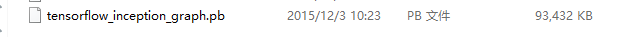checkpoint里存的是各个变量的数值，我们需要重新去构建计算图后可以把checkpoint里的变量值载入进来，就是这个图中对应的变量去checkpoint读取变量值，在tensorflow中还支持对checkpoint做进一步的处理，tensorflow中有一个工具觉freezegraph，就是将checkpoint和计算图给结合起来的一种工具，就是它生成的图是graph和checkpoint变量都在这里面，使用这张图我们就不需要先重建图再去载入变量，直接导入这张图，因为这张图里都是固定的变量，然后就可以直接用这张图里的计算方法去进行处理了~

1.首先载入必要的库：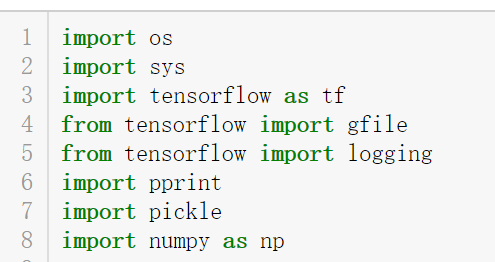（2）定义输入输出文件：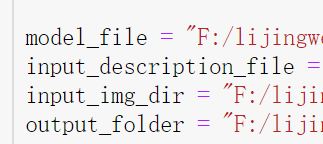（3）因为有大约3万张图片，将所有的图片特征都抽取出放到一个文件夹下是不明智的，所以把这些数据存储在多个文件中，这样每个文件的载入都不会占用太多的时间，所以需要一个参数来确保如何去切分这些数据，即每个子文件中存放多少个数据：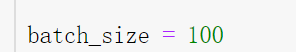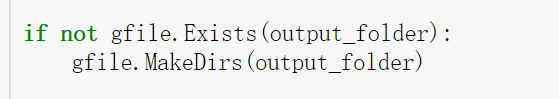（4）解析图片描述文件：